You have recently learned about Python lists, the first mutable data type taught in this beginners course. Before we move on to its immutable counterpart, let's have a closer look what the mutability actually means.

# What Is Mutability and Why Does It Matter

The mutability is a property of a data type telling us whether a value of this data type can change (mutate) in the course of its existence.

Data types can be either immutable or mutable.

Values of immutable data types stay as they were created and cannot change - mutate - in some other value. Immutable types in Python are. e.g., Booleans (`True`, `False`), numbers, strings, tuples.

On the other hand, values of mutable data type can mutate in some other values. Mutable types in Python are. e.g., lists, dictionaries, sets.

Sounds simple, right? Let's see them in detail.

## Immutable Data Types

Let's start with an example of an immutable data type - string. Let's create a value and see what is Python doing:

```>>> example1 = "Hi there!"
```

With this command you created a new string (`"Hi there"`) and assigned it to a variable. Easy! You have done that many times already.

Now, what is actually Python doing is, first, it creates a new value, a string object, and stores in the computer memory and, second, it stores a reference to this value into a variable (`example1`).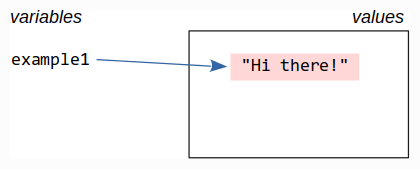What is a reference? In simple words, it is a link to the value stored somewhere in the computer memory. Were and how exactly is stored is not our concern right now.

Let's see what happens when we assign the value held in our variable to another variable like:

```>>> example2 = example1
```

In this assignment, Python takes the reference from the `example1` variable and copies it into `exampled2`. It does not copy the referenced value though.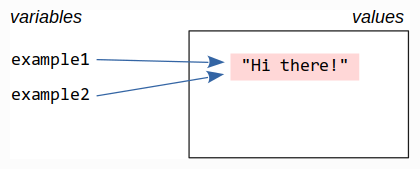How do we check it? Let's compare the actual values first:

```>>> example2 == example1
True
```

Okay, if variables point to the same string object it must be equal, but is it really they the same string object? Let's check it with the Python `is` operator:

```>>> example2 is example1
True
>>>
```

They are. Since the assignment copied a reference to the same string object, both variables refer to the same value (string object).

Now, see what happens if we create a new string rather than a reference to an existing one:

```>>> example3 = "Hi there!"
>>> example3 == example1
True
>>> example3 is example1
False
```

In this case, a new string object is created and its reference is stored in the `example3` variable. The strings to which this variable refers is equal to the one referred by `example1` but it is not the same one (two equal strings occupying two different places in the computer memory).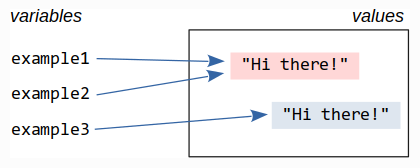All right, this is an interesting detail, but why should we really care?

Well, the nice thing is that with immutable data types we do not need to care. Do you remember what we said before? Immutable values stay as they are and they cannot change (mutate) into something else.

To see the difference, let's repeat the same experiment with a mutable data type.

## Mutable Data Types

We try the same experiment with a mutable data type. Let's create a list and assign it to a variable:

```>>> example1 = [1, 2, 3]
```

We already know what is happening. Python creates a new list object and store its reference into a variable.Note that the list elements are references too, just like the variables.

Let's move to the next step and copy the content of `example1` into a new variable `example2`:

```>>> example2 = example1
>>> example2 == example1
True
>>> example2 is example1
True
```

So far so good, `example2` contains a reference to the same list object as `example1`.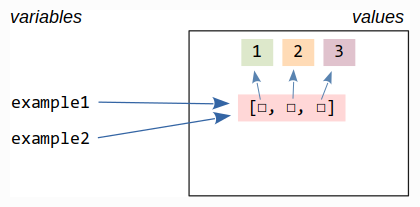Let's create a new list and store in the `example3` variable:

```>>> example3 = [1, 2, 3]
>>> example3 == example1
True
>>> example3 is example1
False
```

All right, nothing new, a new list object is created and its reference is stored in `example3`. This list is equal to the one referenced by `example1` but the referenced objects are different.Now, let's mutate the list referenced by the `example2` variable by appending a new element to the list:

```>>> example2.append(4)
>>> example2
[1, 2, 3, 4]
```

... and check what it is in the other variables

```>>> example1
[1, 2, 3, 4]
>>> example3
[1, 2, 3]
```

All of the sudden, `example1` holds a "different" list than we assigned to it. But we did not touch the `example1` variable!

It will start making sense when we recall that variables in Python hold references to values, list objects in this case, rather than copies of the values.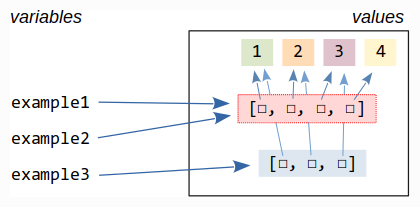The `example1` and `example2` variables refer the same list object and when we change the object through one of the variables it changes also for the other one. And this might be a problem if we do not pay attention with the mutable values.

This is a simple obvious example but in real life this might get less clear. In Python, the references to values are passed not just when we assign values to variables but also when we pass arguments to functions or add elements to list and other composed data types. Or, in other words, Python does not copy the mutable values unless we do it ourselves.

In case of composed data types, we distinguish so called shallow and deep copy. A shallow copy copies just the topmost object leaving the nested references unchanged. A deep copy copies also the nested objects.

You can explicitly request a (shallow) copy, e.g., by using `list(<existing list>)`.

For more details see `copy` documentation

Mutable data types as, e.g, lists are very useful but may lead to undesired side effects and shall be used with care.

There are also cases where mutable values are forbidden such as the keys in dictionaries or sets (worry not, you will learn about them later).

## A Less Obvious Example

Let's continue with a more complex example where mutability may lead to an undesired side effect.

Let's create a 10-by-10 "table" created from lists holding simple character values and a function printing the table in a nice form

```def print_table(table):
for row in table:
print(" ".join(row))

def create_blank_table(n_rows, n_columns, fill_character="."):
row = [fill_character] * n_columns
table = []
for _ in range(n_rows):
table.append(row)
return table

table = create_blank_table(10, 10)

print_table(table)
```

There is an error in the code. Can you find where? If not, don't worry and keep reading ...

The creation looks elegant and simple. The output looks like

``````. . . . . . . . . .
. . . . . . . . . .
. . . . . . . . . .
. . . . . . . . . .
. . . . . . . . . .
. . . . . . . . . .
. . . . . . . . . .
. . . . . . . . . .
. . . . . . . . . .
. . . . . . . . . .``````

The table creation clearly works and produces a list of 10 lists, each of them having 10 elements. Now, let's edit the table and fill it with some new content.

We would like to change elements of the table so that the output looks like this (row 2, column 4 set to `X` and row 5, column 5 set to `O`)

``````. . . . . . . . . .
. . . X . . . . . .
. . . . . . . . . .
. . . . . . . . . .
. . . . O . . . . .
. . . . . . . . . .
. . . . . . . . . .
. . . . . . . . . .
. . . . . . . . . .
. . . . . . . . . .``````

Since you know how to change elements of nested arrays try it yourself

## Conclusion

I hope this chapter helped you better understand what the data type mutability means.

We cannot say that immutable types are always better than the mutable ones, or the other way around. Both have their place in Python.

But remember that multiple references to mutable values may lead to unexpected side effects and strange errors.